Normal view MARC view ISBD view

# Handbook of geometric topology / edited by R.J. Daverman, R.B. Sher.

Material type:TextPublisher: Amsterdam ; New York : Elsevier, 2002Edition: 1st ed.Description: x, 1133 p., USD 305.00 ill. ; 25 cm.ISBN: 0444824324 (alk. paper).Subject(s): Geometric Topology | TopologyDDC classification: 514 Online resources: Table of Contents only | Publisher description
Contents:
Machine generated contents note: 1. Topics in transformation groups -- A. Adem and J.F Davis -- 2. R-trees in topology, geometry, and group theory -- M. Bestvina -- 3. Geometric structures on 3-manifolds -- E Bonahon -- 4. Dehn surgery on knots -- S. Boyer -- 5. Piecewise linear topology -- J.L. Bryant -- 6. Geometric group theory -- J.W. Cannon -- 7. Infinite dimensional topology and shape theory -- A. Chigogidze -- 8. Nonpositive curvature and reflection groups -- M. W Davis -- 9. Cohomological dimension theory -- J. Dydak -- 10. Flows with knotted closed orbits -- J. Franks and M.C. Sullivan -- 11. Nielsen fixed point theory -- R. Geoghegan -- 12. Mapping class groups -- N. V. Ivanov -- 13. Seifert manifolds -- K.B. Lee and E Raymond -- 14. Quantum invariants of 3-manifolds -- W.B.R. Lickorish -- 15. L2-invariants of regular coverings of compact manifolds and CW-complexes -- W. Lack -- 16. Metric spaces of curvature > k -- C. Plaut -- 17. Hyperbolic manifolds -- J.G. Ratcliffe -- 18. Heegaard splittings of compact 3-manifolds -- M. Scharlemann -- 19. Representations of 3-manifold groups -- P.B. Shalen -- 20. Topological rigidity theorems -- C.W. Stark -- 21. Homology manifolds -- S. Weinberger -- Author Index -- Subject Index.
Item type Current location Call number Status Date due Barcode Item holds
Book Chennai Mathematical Institute
General Stacks
516 DAV (Browse shelf) Available 8698
Total holds: 0
##### Browsing Chennai Mathematical Institute Shelves , Shelving location: General Stacks Close shelf browser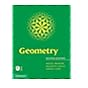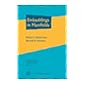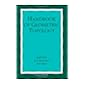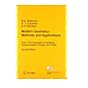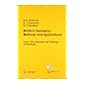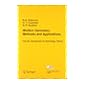516 BRA Geometry / 516 BRE Topology and geometry / 516 DAV Embeddings in manifolds / 516 DAV Handbook of geometric topology / 516 DUB Modern geometry--methods and applications / Part I the geometry of surfaces, transformation groups, and fields. 516 DUB Modern geometry--methods and applications / Part II the geometry and topology of manifolds. 516 DUB Modern geometry--methods and applications / Part III introduction to homology theory.

Includes bibliographical references and indexes.

Machine generated contents note: 1. Topics in transformation groups -- A. Adem and J.F Davis -- 2. R-trees in topology, geometry, and group theory -- M. Bestvina -- 3. Geometric structures on 3-manifolds -- E Bonahon -- 4. Dehn surgery on knots -- S. Boyer -- 5. Piecewise linear topology -- J.L. Bryant -- 6. Geometric group theory -- J.W. Cannon -- 7. Infinite dimensional topology and shape theory -- A. Chigogidze -- 8. Nonpositive curvature and reflection groups -- M. W Davis -- 9. Cohomological dimension theory -- J. Dydak -- 10. Flows with knotted closed orbits -- J. Franks and M.C. Sullivan -- 11. Nielsen fixed point theory -- R. Geoghegan -- 12. Mapping class groups -- N. V. Ivanov -- 13. Seifert manifolds -- K.B. Lee and E Raymond -- 14. Quantum invariants of 3-manifolds -- W.B.R. Lickorish -- 15. L2-invariants of regular coverings of compact manifolds and CW-complexes -- W. Lack -- 16. Metric spaces of curvature > k -- C. Plaut -- 17. Hyperbolic manifolds -- J.G. Ratcliffe -- 18. Heegaard splittings of compact 3-manifolds -- M. Scharlemann -- 19. Representations of 3-manifold groups -- P.B. Shalen -- 20. Topological rigidity theorems -- C.W. Stark -- 21. Homology manifolds -- S. Weinberger -- Author Index -- Subject Index.